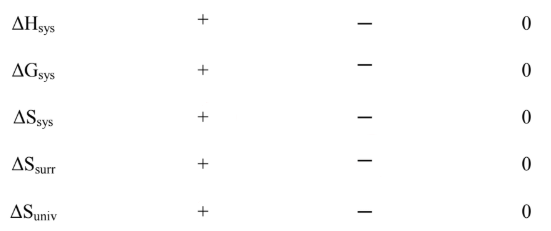# Problem: Potassium nitrate dissolves spontaneously in water, and this process is endothermic. Indicate whether each of the thermodynamic parameters below is positive, negative, or zero. Assume that the external pressure is 1 atm and the temperature of the water remains constant. Circle your answers.A. ΔHsys = (–), ΔGsys = (–), ΔSsys = (–), ΔSsurr = (–), ΔSuniv = (–)B. ΔHsys = (+), ΔGsys = (+), ΔSsys = (+), ΔSsurr = (+), ΔSuniv = (+)C. ΔHsys = (–), ΔGsys = (+), ΔSsys = (–), ΔSsurr = (+), ΔSuniv = (–)D. ΔHsys = (+), ΔGsys = (+), ΔSsys = (–), ΔSsurr = (–), ΔSuniv = (–)E. ΔHsys = (+), ΔGsys = (–), ΔSsys = (+), ΔSsurr = (–), ΔSuniv = (+)

###### FREE Expert Solution

We’re being asked to determine the signs of each of the thermodynamic parameters when potassium nitrate dissolves spontaneously in water.

Potassium Nitrate is an ionic compound and when it dissolves in water, it breaks up into its ions:

KNO3(s) → K+(aq) + NO3-(aq)

For ΔHsys:

Exothermic reactions

lose energy, releases heat, temperature of the surroundings increases
▪ involves bond formation (for phase change: gas → liquid → solid)
ΔH is negative

Endothermic reactions

gain/requires energy, absorbs heat, temperature of the surroundings decreases
▪ involves breaking bonds (for phase change: solid → liquid → gas)
ΔH is positive

88% (32 ratings)###### Problem Details

Potassium nitrate dissolves spontaneously in water, and this process is endothermic. Indicate whether each of the thermodynamic parameters below is positive, negative, or zero. Assume that the external pressure is 1 atm and the temperature of the water remains constant. Circle your answers.A. ΔHsys = (–), ΔGsys = (–), ΔSsys = (–), ΔSsurr = (–), ΔSuniv = (–)

B. ΔHsys = (+), ΔGsys = (+), ΔSsys = (+), ΔSsurr = (+), ΔSuniv = (+)

C. ΔHsys = (–), ΔGsys = (+), ΔSsys = (–), ΔSsurr = (+), ΔSuniv = (–)

D. ΔHsys = (+), ΔGsys = (+), ΔSsys = (–), ΔSsurr = (–), ΔSuniv = (–)

E. ΔHsys = (+), ΔGsys = (–), ΔSsys = (+), ΔSsurr = (–), ΔSuniv = (+)